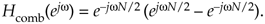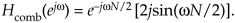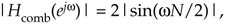# Section G.1. FREQUENCY RESPONSE OF A COMB FILTER

### G 1 FREQUENCY RESPONSE OF A COMB FILTER

The frequency response of a comb filter is Hcomb(z) evaluated on the unit circle. We start by substituting ejw for z in Hcomb(z) from Eq. (7-2), because z = ejw defines the unit circle, givingFactoring out the half-angled exponential e–jwN/2, we have

Equation G-2Using Euler's identity 2jsin(a) = eja – e–ja, we arrive at

Equation G-3Replacing j with ejp/2, we have

Equation G-4Determining the maximum magnitude response of a filter is useful in DSP. Ignoring the phase shift term (complex exponential) in Eq. (G-4), the frequency-domain magnitude response of a comb filter is

Equation G-5with the maximum magnitude being 2.

URL http://proquest.safaribooksonline.com/0131089897/app07lev1sec1AmazonPrev don't be afraid of buying books Next